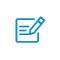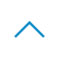# 盘点近期大热对比学习模型：MoCo/SimCLR/BYOL/SimSi

Contrastive loss

def contrastive_loss(self, y,d,batch_size):
tmp= y *tf.square(d)
#tmp= tf.mul(y,tf.square(d))
tmp2 = (1-y) *tf.square(tf.maximum((1 - d),0))
return tf.reduce_sum(tmp +tmp2)/batch_size/2

Easy negative example 比较容易识别，所以相对来说找一些较难的 pair 是有利于训练的。一般可分为：

Offline mining：计算所有的数据的 embedding，然后计算所以 pair 之间的距离判断其难易程度，主要选择 hard 或者 semi-hard 的数据。

Online mining：为每一 batch 动态挖掘有用的数据，将一个 batch 输入到中，得到这个 batch 数据的 embedding，Batch all 的方式还是会计算所有的合理的，Batch hard 偏向于选择距离较大的正样本和距离最小的负样本。

end-to-end：先编码 encoder（可同可不同），然后内积算 loss 再梯度。但是这种方法由于 dictionary size 和 mini-batch 的强耦合性（负例样本对也会为 loss 产生贡献，也会回传梯度），在 batch 大的时候优化难，而在 batch 小的时候，batch 之间的参数会不一样，也就是 GPU 大小限制了模型的性能。

memory bank：把 dictionary size 从 mini-batch 中解耦出来，即先把所有样本的特征保存下来 bank，然后每次随机采样，再梯度 query 的 encoder 的参数。但是这样只有当所有 key 被 sample 完以后才会更新 memory bank，不同的 key 在和 query 是不一致的和滞后的，因为每一次 sample encoder 都会更新虽有 memory bank 后面也加入了 momentum，但是是针对 sample 来的，在更新 memory bank 时会保留一部分上一轮的特征值。

MoCo：是以上两者的融合版本，将 dictionary 作为一个 queue 进行维护当前的negative candidates pool，且它是改成了 queue 的动态更新机制，每 sample 一个 batch key（所以一个 trick 就是会使用 Shuffling BN，打乱再 BN），进队后相对于一些最早进入队列的 mini-batch 对应的 key 进行出队操作，这样保证一些过时的、一致性较弱的 key 可以被清除掉。这样就同样是解耦，K 是队列长度，K 可以设置很大，同时更新也不会有问题。

def _momentum_update_key_encoder(self):
"""
key encoder的Momentum update
"""
for param_q, param_k in zip(self.encoder_q.parameters(), self.encoder_k.parameters()):
param_k.data = param_k.data * self.m + param_q.data * (1. - self.m)

def _dequeue_and_enqueue(self, keys):
"""
完成对队列的出队和入队更新
"""
# 在更新队列前得到keys
keys = concat_all_gather(keys)#合并所有keys

batch_size = keys.shape

ptr = int(self.queue_ptr)
assert self.K % batch_size == 0  # for simplicity

# 出队入队完成队列的更新
self.queue[:, ptr:ptr + batch_size] = keys.T
ptr = (ptr + batch_size) % self.K  # 用来移动的指针

self.queue_ptr = ptr

def forward(self, im_q, im_k):
# 计算query features
q = self.encoder_q(im_q)  # queries: NxC
q = nn.functional.normalize(q, dim=1)

# 计算key features
self._momentum_update_key_encoder()  # 用自己的来更新key encoder

# 执行shuffle BN
im_k, idx_unshuffle = self._batch_shuffle_ddp(im_k)

k = self.encoder_k(im_k)  # keys: NxC
k = nn.functional.normalize(k, dim=1)

# 还原shuffle
k = self._batch_unshuffle_ddp(k, idx_unshuffle)

# 计算概率
# positive logits: Nx1
l_pos = torch.einsum('nc,nc->n', [q, k]).unsqueeze(-1) #用爱因斯坦求和来算sum
# negative logits: NxK
l_neg = torch.einsum('nc,ck->nk', [q, self.queue.clone().detach()])

# logits: Nx(1+K)
logits = torch.cat([l_pos, l_neg], dim=1)

# 平滑softmax的分布，T越大越平
logits /= self.T

# labels是正例index
labels = torch.zeros(logits.shape, dtype=torch.long).cuda()

# 出队入队更新
self._dequeue_and_enqueue(k)

return logits, labels

https://arxiv.org/abs/1911.05722

https://github.com/nakaizura/Source-Code-Notebook/tree/master/MoCo

SimCLR
MoCo 强调 pair 对的样本数量对对比学习很重要，SimCLR 认为构建负例的方式也很重要。先说结论：

https://github.com/aleju/imgaug

https://arxiv.org/abs/2002.05709

MoCo v2

SimCLR v2

BYOL

online 网络参数使用 L2 的梯度进行更新，而 target 网络直接通过 online 的 momentum 得到，这里 target 的就充当了之前负样本的功能。

BYOL和MoCo、SimCLR的区别
MoCo、SimCLR 更偏向于问这两张图片之间有何差异？
BYOL 可能是在问这张图片与这些图片的平均有什么差异？

https://arxiv.org/abs/2006.07733

SimSiam

# Algorithm1 SimSiam Pseudocode, Pytorch-like
# f: backbone + projection mlp。f是backbone+projection head部分组成
# h: prediction mlp
for x in loader: # load a minibatch x with n samples
x1, x2 = aug(x), aug(x) # random augmentation，随机增强后的x1和x2
#分别做两次投影操作
z1, z2 = f(x1), f(x2) # projections, n-by-d
p1, p2 = h(z1), h(z2) # predictions, n-by-d
#计算不对称的两个D得到loss L
L = D(p1, z2)/2 + D(p2, z1)/2 # loss
L.backward() # back-propagate，反向传播
update(f, h) # SGD update，梯度更新

def D(p, z): # negative cosine similarity
p = normalize(p, dim=1) # l2-normalize
z = normalize(z, dim=1) # l2-normalize
return -(p*z).sum(dim=1).mean()

https://arxiv.org/abs/2011.10566扫码入群返回顶部